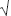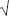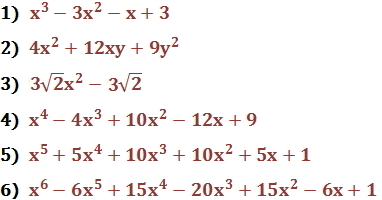Expand Polynomials

Expand Polynomials

A polynomial is expanded if no variable appears within parentheses and all like terms have been combined.

To expand a polynomial, multiply its factors (often by using the distributive property) or perform the indicated operations.  Then combine all like terms.

Example

Expand the following expression.

3x(4 - x) + (2x - 5)2

Remembering the order of operations, we take care of exponents before multiplication.

Because (2x - 5)2 = (2x - 5)(2x - 5), recall that we can use the FOIL method to multiply this out, then combine like terms:

(2x - 5)(2x - 5) = 4x2 - 10x - 10x + 25

= 4x2 - 20x + 25

Now we have:

3x(4 - x) + (4x2 - 20x + 25)

Multiply the 3x by (4 - x) using the distributive property:

(12x - 3x2) + (4x2 - 20x + 25)

Now all that's left is to add the polynomials by combining like terms.  Remember, when complete, the polynomial is written in standard form, so the exponents are in descending order:

(12x - 3x2) + (4x2 - 20x + 25) = -8x + x2 + 25 = x2 - 8x + 25

Practice

1)    (x + 1)(x - 1)(x - 3)

2)    (2x + 3y)2

3)    (3x3)(6x6)

4)    (x - 2x + 3)2

5)    (x + 1)5

6)    (x - 1)6